﻿ New atomic structure (Beryllium,Boron,Carbon)

# Bohr's New Atomic and Molecular Structure

## Present quantum mechanics stops development of all science.

### [ Molecular biological technology has made NO progress since 1970s. ]

(Fig.A-1) Development of molecular technology has stopped since 1970s.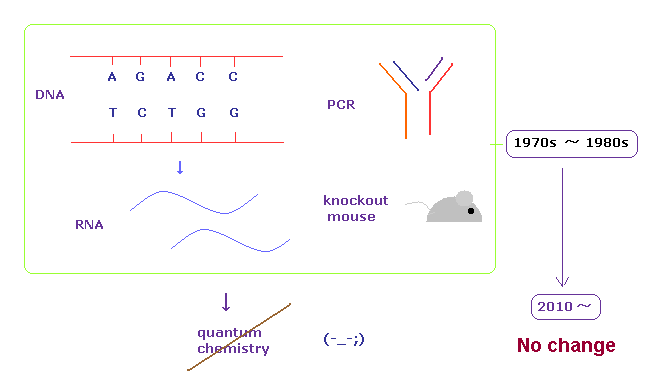You may often hear the news such as " molecular biology is progressing day by day. ".
But for example, we cannot deal with serious diseases such as cancer and various functional disorders at all.
The present molecular biological technologies dealing with DNA, RNA, PCR, cultured cells and knockout mice have been established in 1970s - 1980s.

Unfortunately, even in the 21st century, these old molecular technologies are still playing the leading role in leading-edge fields.
This is very strange.
These serious situations are caused by the fact that the unrealistic quantum mechanics ( chemistry ) can NOT be applied to molecuar technology at all.

(Fig.A-2) All living things can be made directly from elements themselves.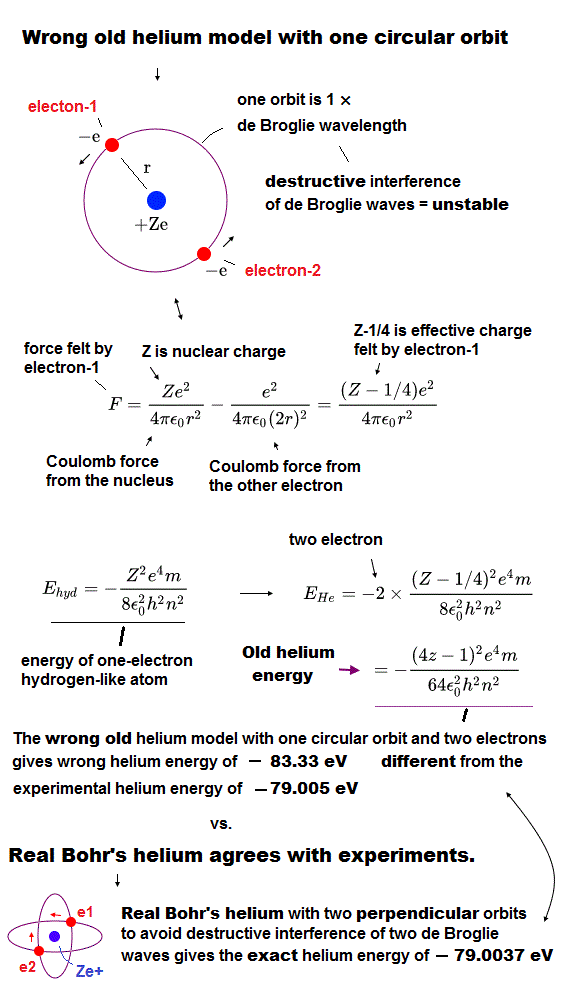As you know, originally, all living things on the earth were just separated elements with no life.
And taking extremely long time, the nature on the earth has made all living organisms from elements.

This means it is possible that we can produce all living things from separated elements, and control them on the earth with some knowledge.
But now, we cannot do it ( even protist ) at all, and have made NO progress in atomic levels.

Comparing with very complicated human body, atomic structure is very simple, composed of electrons and nuclei.
This indicates various motion patterns of each electron determine and control various biological functions.
So we must discard problematic uncertainty principle (= Shut up and calculate ! ) as early as possible.

(Fig.A-3) Present science is going toward unrealistic and illusory fields.In some science news and websites, we often see the news dealing with expanding universe, black holes, quantum computer, another dimensions and spin electronics.
Actually, all developments of our science have stopped due to these unreal and unuseful things.
And special relativity is wrong, so standard model, string theory, and general relativity can NEVER be true theories.

We see the news such as Higgs has been found extremely many times.
But they NEVER explain the difference between infinite Higgs fields and "ether", and the concrete mechanisms of how each element can get masses ( "resistance" is contradictory , as shown on this page ).
So ordinary people and students with strong curiosity are very frustrated, I think.

## Realistic Bohr's two-electron and three-electron atoms perfectly agree with experimental energy values.

### [ Two electrons' de Broglie wave orbits must be perpendicular to each other to avoid destructive interference.  → good agreement with experimental energy values. ]

(Fig.1) "Real" two and three electron atomic structure.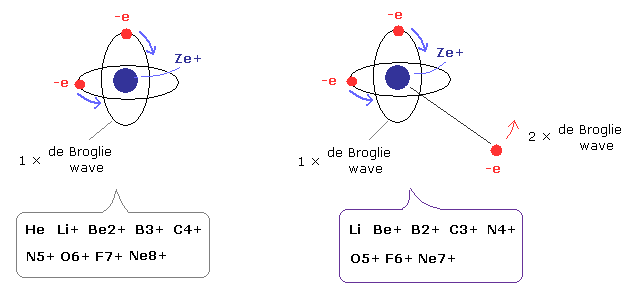Successful new Bohr model can perfectly predict correct atomic energy values of not only one-electron hydrogen-like atoms but also two-electron, three-electron atoms and ions with No contradiction ( unlike unscientific quantum mechanics ) as shown in this Top page.

In this new successful Bohr model, the two electrons of the helium atom (He) are moving on the orbits of just one de Broglie's wavelength which are perpendicular to each other.

Because many experiments showed that an electron causes de Broglie wave which interferes with each other destructively and constructively like other ordinary waves.

When the two electrons' orbits of 1 × de Broglie wavelength overlap each other on the same circular orbit like old Bohr's helium model, the opposite wave phases of their 1 × de Broglie wave orbits cancel each other out (= crest and trough of electron's 1 × de Broglie wave orbit interfere with each other destructively ), and resultantly kicking out electrons, and become unstable.

This model can explain the phenomena of Pauli exclusion principle correctly and realistically ( instead of quantum mechanical unrealistic Pauli exchange energy ), because there is no space for the third electron ( of 1 × de Broglie wave ) to enter this new two-electron Bohr helium atom without causing destructive interference (= real Pauli principle repulsion origin ) with other de Broglie wave orbits.

Surprisingly, this new atomic structure of Bohr's helium can predict exact experimental energy values in all other two and three electron atoms ( ions ).

(Table 1) Good agreement between prediction and experimental values in two-electron atoms and ions.
Atoms r1 (MM) one wavelength old circular orbit Predicted values (eV) Experiment energy (eV)Error (eV)
He 3074.0 1.000000 -83.335-79.0037 -79.0051 0.001
Li+ 1944.5 1.000000 -205.78-198.984 -198.093-0.89
Be2+ 1422.0 1.000000 -382.66-373.470 -371.615-1.85
B3+ 1121.0 1.000000 -613.96-602.32 -599.60-2.72
C4+ 925.0 1.000000 -899.67-885.6 -882.1-3.50
N5+ 788.0 1.000000 -1239.8-1223.3 -1219.1-4.20
O6+ 685.3 1.000000 -1634.38-1615.44 -1610.70-4.74
F7+ 607.3 1.000000 -2083.3-2062.0 -2057.0-5.00
Ne8+ 544.5 1.000000 -2586.7-2563.0 -2558.0-5.00

(Fig.2) Real Two-electron Atomic Model ( He, Li+, Be2+, B3+, C4+ ... )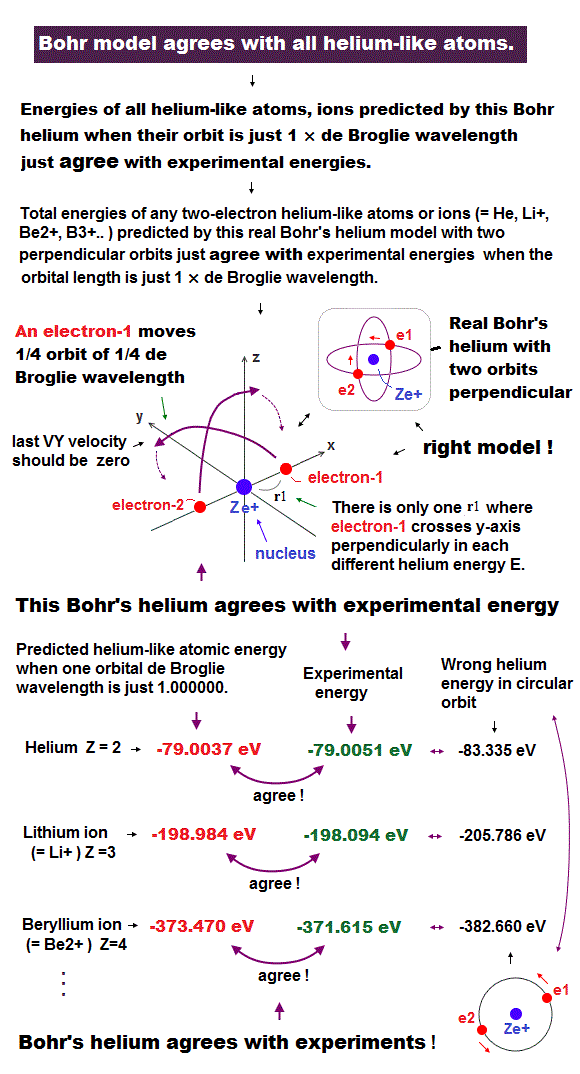The calculated results of Table 1 (= predicted ground-state energy values by this new helium model with two perpendicular orbits showed good agreement with experimental atomic and ions' energy values ) clearly show that the Fig.2 atomic model is true.

As positive charges of ions are increased, the errors (= disagreement between predicted and experimental energies) become slightly bigger, probably because the stronger Coulomb electric interactions between highly-charged ions tend to make electrons' motions and experimental energies a little more unstable.

## Old circular orbits of old Bohr's helium model gives wrong energy.

(Fig.3) Circular orbit of two-electron atoms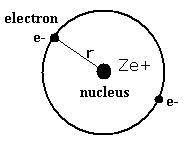Old Circular orbit of Table 1 means the two electrons are at the opposite sides on the same one orbit like Fig.3.
Of course, Fig.3 model is wrong, because the opposite wave phases of their 1 × de Broglie waves cancel each other out by destructive interference.

Actually, this old Bohr's helium circular orbits (= two electrons exist in the same one circular orbits on the opposite sides of the nucleus ) gives the wrong helium's ground state energy (= -83.33 eV,  this p.3-table.3 ) which is lower than the experimental helium's ground state energy (= -79.005 eV )

## Three-electron atoms and ions also show good agreement with experimental energy values.

(Table 2) Good agreement between prediction and experimental values in three-electron atoms and ions.
Atoms r1 (MM) one wavelength Predicted values (eV) Experiment energies (eV)Error (eV)
Li 1949.0 1.000000 -203.033 -203.480 0.47
Be+ 1427.0 1.000000 -388.785 -389.826 1.04
B2+ 1125.0 1.000000 -635.965 -637.531 1.56
C3+ 928.0 1.000000 -944.46 -946.57 2.11
N4+ 790.5 1.000000 -1314.25 -1317.01 2.76
O5+ 688.0 1.000000 -1745.70 -1748.82 3.12
F6+ 609.4 1.000000 -2237.60 -2242.21 4.61
Ne7+ 546.0 1.000000 -2791.15 -2797.12 5.97

(Fig. 4) Three electron atomic model.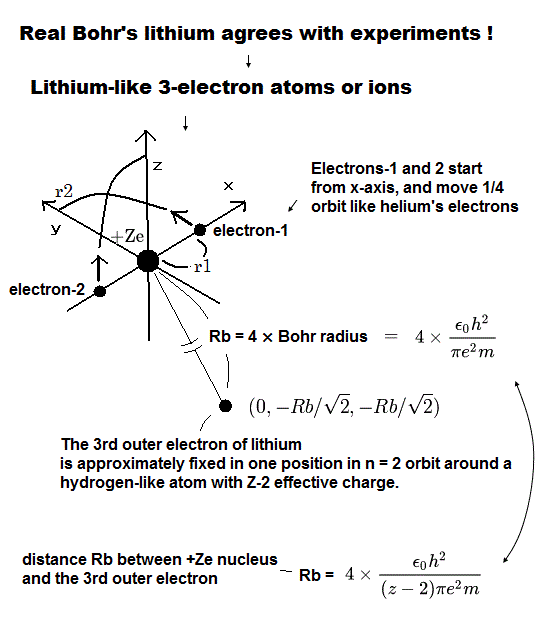In the three-electron atomic model, only an outer 2s electron (= 2 × de Broglie wavelength orbit or n = 2 ) is distanced from other inner 1s electrons (= 1 × de Broglie wavelength orbit or n = 1 ), so we can approximately think this outer electron orbits around an inner central positive charge with effective charge of Z-2 (= Z of nuclear positive charge of atoms minus two inner electrons ) like hydrogen-like atoms.

As a result, the approximate radius of the outer 2S electron of three-electron lithium atom (= Li ) becomes Bohr radius × 4 (= n2 where n = 2 ) in the lithium atom ( Z = 3, hence the effective positive central charge becomes Z-2 = 1 ).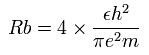In other ions, divide the radius by (Z-2). Z means atomic number.
Because we consider the two inner 1S electrons are very close to the Z nucleus.
So the 2S electron is going around the +(Z-2) nucleus as if hydrogen-like atom.
In larger atoms, using this rough approximation in outer orbit is the reason why slightly bigger errors are caused in larger three-electron ions as shown in Table 2.
( But in all three-electron atoms and ions, this new atomic model is in very good agreement with experimental energy values. )

Here we show experimental values of ionization energies (eV) of various atoms ( this or this ).

(Table 3) Ionization energies of the elements (eV).
(Experimental values.)
Atomic Name 1st 2nd 3rd 4th 5th 6th
Helium (He) 24.58741 54.41778 - - - -
Lithium (Li) 5.39172 75.64018 122.45429 - - -
Beryllium (Be) 9.32270 18.21116 153.89661 217.71865 - -
Boron (B) 8.29803 25.15484 37.93064 259.37521 340.22580 -
Carbon (C) 11.26030 24.38332 47.88780 64.49390 392.08700 489.99334

The sum of the last two large values (= red values ) of Table 3 means the experimental total energy values of the two electron atoms (ions) in Table 1.
And the sum of the last three values (= two red + one green values ) means the experimental total energy values of the three electron atoms (ions) in Table 2.

The ionization energies of 1S electrons ( red values of Table 3 ) are much bigger than other ionization energies.
This means that in various atoms, only two "1S" electrons are much closer to the nucleus than other electrons (= only inner two electrons are moving in the smaller 1 × de Broglie wavelength orbits, and the 3rd electron is moving in outer larger 2 × de Broglie wavelength orbit ).

These experimental energy values prove that electron's orbital length must be equal to an integer times de Broglie waves to avoid destructive interference of de Broglie wave.

## Old Bohr's helium model with one circular orbit with two electrons was false.

Old Bohr's circular orbits successfully explained experimental energy values of all hydrogen-like atoms and ions considering experimentally verified de Broglie wave interference.

But calculation of two-electron helium atomic orbits by considering destructive interference between two moving electrons' de Broglie wave orbits was impossible in old 1920s without modern computers.

Here we can easily calculate the energy value of the simple circular orbit of old Helium atomic model (= this old model's energy value disagreed with the experimental helium ground state energy ) as shown in Fig.3' and Table 1.

Due to the lack of modern computers in 1920s, this old Bohr's helium circular obit was the only model which could be calculated with a pen and paper without calculations by computers.  ← This lack of modern computer in 1920s is the reason why physicists had to reluctantly accept unrealistic quantum mechanics.

Quantum mechanical illegitimate approximate methods just choose false solutions manipulating freely-adjustable parameters without solving unsolvable Schrödinger equations.  ← Quantum mechanics has No power to predict any multi-electron atoms such as helium.

(Fig.3') Old Bohr's circular helium↑ This circular orbit of old Helium-like atomic model gives wrong energies due to ignoring destructive interference of two electrons' de Broglie wave orbits.

Two electrons are moving on just the opposite sides of the Ze+ (= Z = 2 in Helium atom ) positive nucleus in the same circular orbit whose circumference is 1 × de Broglie wavelength.

This old Bohr's helium model gives the wrong helium ground state energy (= -83.33 eV,  this p.3-table.3 ) which is lower than the experimental helium ground state energy (= -79.005 eV,  this p.4,  this p.9 )

Equating the centrifugal force to the Coulomb force, we have
(Eq.2)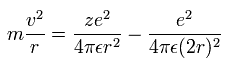where r is the circular orbital radius (meter), m is the electron mass, e is the electron's charge.
And "z" is atomic number.

↑ From the upper Coulomb force relation, you will easily find each electron is orbiting arount the central effective positive charge ( Z - 1/4,  Helium nculear charge Z = 2, hence, the effective central positive charge becomes 2-1/4 = 7/4 ) like a hydrogen-like atom.

As a result, this old circular helium model gives the wrong total energy = -13.6 eV (= hydrogen ground-state energy ) × Z2 (= effective Z = 7/4 ) × 2 = -83.3 eV.

The right-hand side of Eq.2 consists of Coulomb attractive force between each electron and nucleus (= 1st term ) and repulsion between two electrons (= 2nd term ).

The circular orbital length is supposed to be an integer (n) times de Broglie wavelength of the electron.
(Eq.3)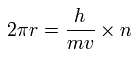where h/mv is the de Broglie's wavelength.

The total energy E equal to the sum of the kinetic and the Coulomb potential energies of the two electrons in circular Helium model becomes
(Eq.4)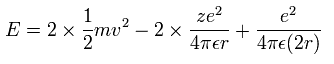Solving the above three equations (= Eq.2-4 ), the total energy E becomes,
(Eq.5)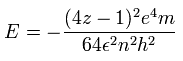Substituting the atomic number into z ( z = 2 in Helium atom ), n=1 (= ground state ), and other physical constants, and converting the energy value from joules to eV, we get the results of Table 1 (= old circular orbit ).

For example, if Helium atom is a circular orbit like Fig.3', this total ground state energy becomes -83.33 eV which is lower than the actual Helium energy (= -79.005 eV, which is the sum of first and second ionization energies of Helium, 79.005 eV = 24. 5874 eV + 54.417 eV in this CRC values ).

So this old Helium model of circular orbit with two electrons on the opposite sides of a nucleus gives larger (= wrong ) ionization energies than the experimental results.

Because a single 1 × de Broglie wavelength orbit includes two electrons, which put their waves into disorder by destructive interference of two electrons' de Broglie waves.

True two-electron Helium-like atoms must have two orbits crossing perpendicularly in order to avoid destructive interference of two de Broglie waves.

## New Bohr's realistic model's prediction agrees with experimental energy values of two-electron atoms and ions.

(Fig.2) Two-electron Atomic Model ( He, Li+, Be2+, B3+, C4+ ... )In this section, we actually compute the ground state energy of Li+, Be2+ and B3+ using the same method as new Bohr's helium model.
See the Top page (He) about the detailed computing methods.
Here we use the next sample JAVA program ( or simple C language ).

In this program, we use the new units of
(Eq.6)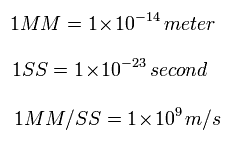From Eq.6, the acceleration is
(Eq.7)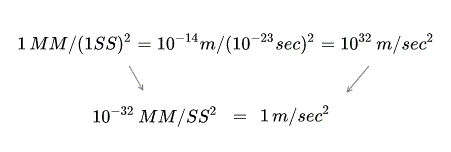In this program, we first input the atomic number Z (= 2, 3, 4, 5, ... or 10 ) .
Next we input the initial x-coordinate r1 (in MM) of electron 1 (see Fig.2), and the absolute value of the total energy E (in eV) of two-electron atoms.
At intervals of 1 SS, we compute the Coulomb force among the two electrons and the nucleus.
When the electron 1 is at (x, y, 0), the electron 2 is at (-x, 0, y).
So the x and y components of the acceleration (MM/SS2) of the electron 1 is,

(Eq.8) x and y components of the acceleration.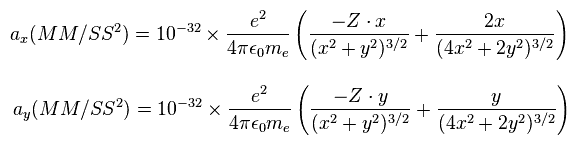where the first term is by the Coulomb force between the nucleus and the electron 1, and the second term is by the force between the two electrons.

In light atoms such as helium and lithium, we use the reduced mass, rm =1/2 × (2me × nucleus)/(2me + nucleus) except when the center of mass is at the origin (= of course, even if you use the ordinary electron's mass instead of reduced mass, you can get almost the same correct energy values ).

We also calculate de Broglie's wavelength of the electron from the velocity ( λ = h/mv ) at intervals of 1 SS.
The number of that wave ( λ in length ) included in that short movement section ( the sum of them is WN ) is,

(Eq.9) Number of de Broglie's wave included in the short movement segment for 1SS.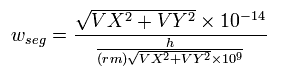where ( VX, VY ) are the velocity of the electron 1 ( in MM/SS ), the numerator is the movement distance (in meter) for 1 SS. the denominator is de Broglie's wavelength ( in meter ).

Here, the estimated electron's orbit is divided into more than one million short segments for the calculation.
When the electron 1 has moved one quarter of its orbit and its x-coordinate is zero, this program checked the y-component of the electron 1 velocity ( last VY ).
When the last VY is zero, two electrons are periodically moving around the nucleus on the same orbitals.

So, only when -0.0001 < last VY < 0.0001 ( MM/SS ) is satisfied, the program displays the following values on the screen, r1, VY, preVY ( VY 1 SS ago ), and (mid)WN ( total number of de Broglie's waves contained in one quarter of the orbit ).
( "Wrong Ene" means the total energy of circular orbits given by Eq.5. )

### [ Lithium ion ( Li+ ) computing results show good agreement between prediction and experimental energy values. ]

(Table 4) Prediction agrees with experimental Li+ ground state energy (= -198.093 eV ).
Prediction (eV) r1 (MM) 1/4 wavelength one wavelength
-194.0 1995.0 0.253191 1.012764
-196.0 1974.0 0.251895 1.007580
-198.0 1954.0 0.250620 1.002480
-198.984   ← 1944.5 0.250000 1.000000
-200.0 1935.0 0.249364 0.997456
-202.0 1916.0 0.248126 0.992504
-204.0 1897.5 0.246907 0.987628

Table 4 shows the results of r1 (= initial electron-1 x-coordinate ) and WN (= number of de Broglie wavelength included in 1/4 of the orbit ) in which the electron-1's last velocity VY is zero at various energy levels of Li+.

So when the total energy of Li+ is -198.984 eV, the number of de Broglie's waves contained in one orbits becomes just 1.000000.
The experimental value of Li+ ground state energy is -198.093 eV.
The error is only -0.89 eV ( 0.4 %).

This very good agreement between predicted energy and actual experimental values shows that also in the lithium ion ( Li+ ), the orbital planes of the two electrons are almost perpendicular to each other to avoid destructive interference of two de Broglie waves.

### [ Beryllium ion ( Be+ ) computing results show good agreement between prediction and experimental energy values. ]

(Table 5) Prediction shows good agreement with experimental Be2+ ground state energy (= -371.615 eV ).
Prediction (eV) r1 (MM) 1/4 wavelength one wavelength
-367.0 1447.0 0.252194 1.008776
-369.0 1439.0 0.251510 1.006040
-371.0 1431.0 0.250831 1.003324
-373.47   ← 1422.0 0.250000 1.000000
-375.0 1416.0 0.249490 0.997960
-377.0 1409.0 0.248827 0.995308
-379.0 1401.0 0.248169 0.992676

Table 5 shows the results of r1 and WN (= number of de Broglie wavelength included in 1/4 of the orbit ) in which electron's last velocity VY is zero at various energy levels of Be2+.

So when the total energy of Be2+ is -373.47 eV, the number of de Broglie's waves contained in one orbits becomes just 1.000000.
The experimental value of Be2+ ground state energy is -371.62 eV.
The error is only -1.85 eV ( 0.4 %).

This very good agreement between predicted energy and actual experimental values shows that also in Beryllium Be2+ ion, the orbital planes of the two electrons are almost perpendicular to each other to avoid destructive interference of two de Broglie waves.

### [ Boron ion ( B3+ ) computing results show good agreement between prediction and experimental energy values. ]

(Table 6) Prediction shows good agreement with experimental B3+ ground state energy (= -599.60 eV ).
E (eV) r1 (MM) WN WN x 4
-596.0 1133.0 0.251322 1.005288
-598.0 1129.0 0.250902 1.003608
-600.0 1125.0 0.250482 1.001928
-602.32 1121.0 0.250000 1.000000
-604.0 1118.0 0.249652 0.998608
-606.0 1114.0 0.249240 0.996960
-608.0 1110.0 0.248830 0.995320

Table 6 shows the results of r1 and WN (= number of de Broglie wavelength included in 1/4 of the orbit ) in which the electron's last velocity VY is zero at various energy levels of B3+.

So when the total energy of B3+ is -602.32 eV, the number of de Broglie's waves contained in one orbits becomes just 1.000000.
The experimental value of B3+ ground state energy is -599.60 eV.
The error is only -2.72 eV ( 0.4 %).

This very good agreement between predicted energy and actual experimental values shows that also in the Boron B3+ ion, the orbital planes of the two electrons are almost perpendicular to each other to avoid destructive interference of two de Broglie waves.

## New Bohr's realistic model's prediction also agrees with experimental energies of all three-electron atoms and ions.

(Fig.4) Three-electron atomic model with two perpendicular inner 1s orbits + one outer 2s orbitNext we compute three-electron atoms (ions).

For example, lithium atom has two electrons in two inner 1S orbits, and one electron in outer 2S orbit.

The two electrons of 1S state are attracted to the 3e+ nucleus strongly, so they are much closer to the nucleus than the 2S electron.

The experimental fact that the lithium ionization energy of only the outer electron (= 5.39 eV ) is much smaller than the ionization energies of the other two inner electrons (= 75.6 eV and 122.4 eV ) shows only the outer 2s orbit is far away from the nucleus in the large 2 × de Broglie wavelength orbit (= n = 2 ), other inner electrons are in the smaller 1 × de Broglie wavelength orbit (= n = 1), as shown in this 5th apragraph.

So approximately, we can suppose the outer solitary 2S electron is moving around the effective central positive charge e+ ( +3e - 2e = +e ) on the circular orbit of the two de Broglie's wavelength.
(This means that we can use the equations of the hydrogen atom in 2S electron approximately.)
2S electron of the ions with atomic number Z is considered to be rotating around effective charge Z-2 nucleus like hydrogen-like atoms.

According to the Bohr model (= equal to quantum mechanical hydrogen energies ), the radius (Rb) of the two de Broglie's wavelength orbit (= n = 2 ) and the effective central charge Z=1 is 4 × Bohr radius, as follows,
(Eq.10)This Rb is 2.1167 × 10-10 meter ( = 21167 MM where 1 MM = 1 × 10-14 meter ) .

And the energy level of n =2 becomes, (using the energy levels of the hydrogen atom)
(Eq.11)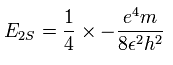The two electrons of 1S state are avoiding 2S electron, so we approximately fix the position of the outer 2S electron at ( 0, - Rb / √2, - Rb / √2 ) as shown in Fig.4.
(Fig.4 shows the relative positions, But actually, this 2S electron is rotating around the nucleus slower than the 1S electrons.)

Like two-electron ions with two perpendicular orbits avoiding destructive interference of two de Broglie wave orbits, when the electron 1 is at (x, y, 0) in xy plane, the electron 2 is at ( -x, 0, y ) in xz plane. So the distances among the particles are,
(Eq.12)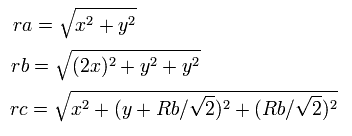where ra is the distance between the electron 1 and the nucleus,
rb is between two 1S electrons, and rc is between the electron 1 and 2S electron, respectively.

The x component of the acceleration (m/sec2) of the electron 1 is ( Li case ),
(Eq.13)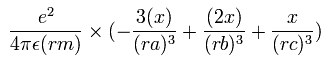In the same way, the y component of the acceleration (m/sec2) of the electron 1 is,
(Eq.14)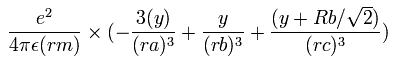Then we compute the number of de Broglie's waves contained in one quarter of the 1S orbit like two-electron ions.

In this program, we first input atomic number Z = 3, 4, 5, 6, 7, 8, 9, or 10. ( Li Z=3, Ne7+ Z=10 )
Next we input the initial x-coordinate r1 (in MM) of electron 1, and the absolute value of the total energy E (in eV) of three-electron atoms (ions).
From the inputted values, this program outputs the y component of electron 1 velocity after a quarter of its orbit, and WN (the number of de Broglie's waves included in one quarter of the orbital).
"Rbb" means the radius (MM) of 2S electron.

### [ Lithium ( Li ) computing results show good agreement between prediction and experimental energy values. ]

(Table 7) Prediction shows good agreement with experimental Li ground state energy (= -203.480 eV )
Prediction (eV) r1 (MM) 1/4 wavelength one wavelength
-197.0 2010.0 0.253889 1.015556
-199.0 1990.0 0.252579 1.010316
-201.0 1969.0 0.251290 1.005160
-203.033   ← 1949.0 0.250000 1.000000
-205.0 1930.0 0.248770 0.995080
-207.0 1911.0 0.247538 0.990152
-209.0 1892.0 0.246324 0.985296

Table 7 shows the results of r1 and WN (= number of de Broglie wavelength included in 1/4 of the inner orbit ) in which the inner electron-1's last velocity VY is zero at various energy levels of Li.

So when the total energy of Li is -203.033 eV, the number of de Broglie's waves contained in one 1S orbit becomes just 1.000000.
The experimental value of Li energy is -203.48 eV.
The error is only 0.447 eV ( 0.2 %).

This very good agreement between predicted energy and actual experimental values shows that also in Lithium neutral atom (= Li ), the orbital planes of the two inner electrons are almost perpendicular to each other to avoid destructive interference of two de Broglie waves.

### [ Beryllium ion ( Be+ ) computing results show good agreement between prediction and experimental energy values. ]

(Table 8) Prediction shows good agreement with experimental Be+ ground state energy (= -389.826 eV ).
Prediction (eV) r1 (MM) 1/4 wavelength wavelength
-383.0 1450.0 0.251968 1.007872
-385.0 1442.0 0.251282 1.005128
-387.0 1434.0 0.250602 1.002408
-388.785   ← 1427.0 0.250000 1.000000
-390.0 1422.0 0.249593 0.998372
-392.0 1415.0 0.248926 0.995704
-394.0 1407.0 0.248265 0.993060

Table 8 shows the results of r1 and WN (= number of de Broglie wavelength included in 1/4 of the inner orbit ) in which the inner electron-1's last velocity VY is zero at various energy levels of Be+.

So when the total energy of Be+ is -388.785 eV, the number of de Broglie's waves contained in one 1S orbit becomes just 1.000000.
The experimental value of Be+ energy is -389.826 eV.

This very good agreement between predicted energy and actual experimental values shows that also in Beryllium Be+ ion, the orbital planes of the two inner electrons are almost perpendicular to each other to avoid destructive interference of two de Broglie waves.

### Various atoms and molecular model (= old version ).

Let's stop believing vague wave function "blindly" to develop "real" science.
Schrodinger wave function can NOT give the clear model of helium and other atoms.
( This is a main reason why the wave function gives only probability and many-world concept becomes dominant. )
So from the beginning, the quantum mechanical helium, which we can NOT understand, does NOT stand on the same stage as Bohr model.2010/7/28 updated. Feel free to link to this site.